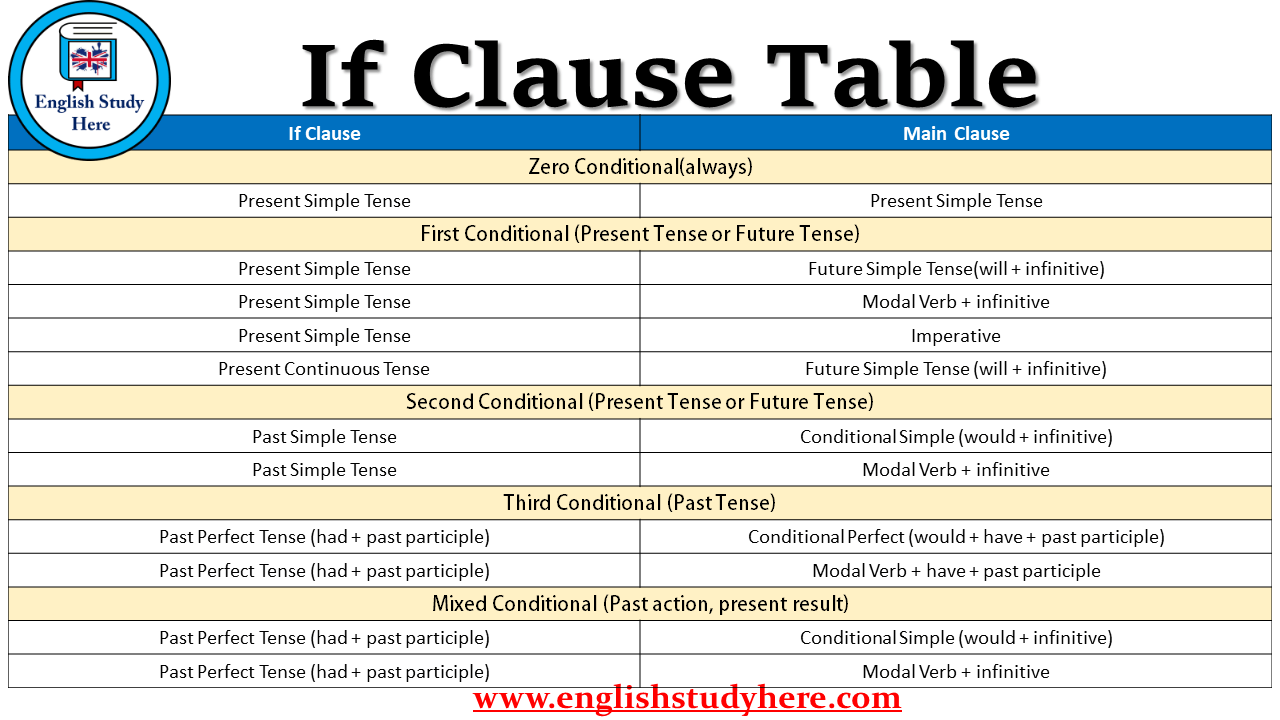If Clause Table – Conditionals Table

If Clause Table, Conditionals Table, Conditionals and IF clauses;If Clause Main Clause Zero Conditional(always) Present Simple Tense Present Simple Tense First Conditional (Present Tense or Future Tense) Present Simple Tense Future Simple Tense(will + infinitive) Present Simple Tense Modal Verb + infinitive Present Simple Tense Imperative Present Continuous Tense Future Simple Tense (will + infinitive) Second Conditional (Present Tense or Future Tense) Past Simple Tense Conditional Simple (would + infinitive) Past Simple Tense Modal Verb + infinitive Third Conditional (Past Tense) Past Perfect Tense (had + past participle) Conditional Perfect (would + have + past participle) Past Perfect Tense (had + past participle) Modal Verb + have + past participle Mixed Conditional (Past action, present result) Past Perfect Tense (had + past participle) Conditional Simple (would + infinitive) Past Perfect Tense (had + past participle) Modal Verb + infinitive

One thought on “If Clause Table – Conditionals Table”

•December 23, 2018 at 12:53 pm